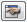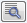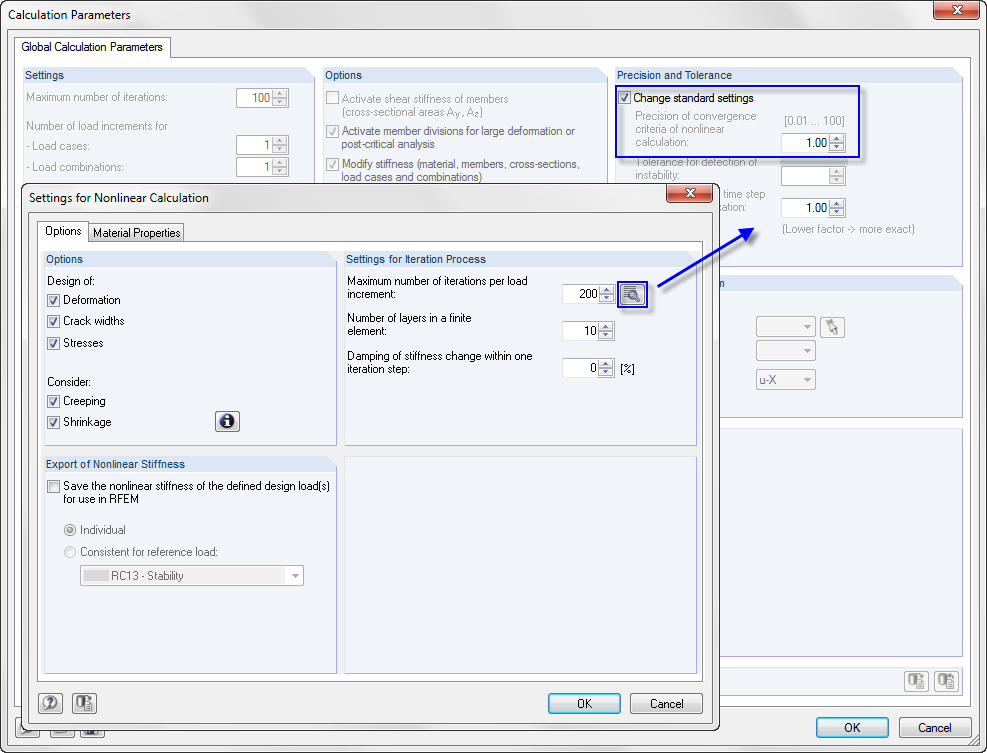# RF-CONCRETE Surfaces – Online Manual Version 5

Online manuals, introductory examples, tutorials, and other documentation.

# 2.8.2.4 Convergence criteria

#### Convergence criteria

In solving the nonlinear equations, two convergence criteria are considered. The iteration step is deemed to be completed once a convergence criterion is fulfilled. The first convergence criterion observes how the diagonal components of the material's stiffness matrix change. A convergence is reached when the stiffness matrix of the material has stabilized for all finite elements.

where

 stiffness matrix of the material from the previous iteration step stiffness matrix of the material in the current iteration step ε desired precision (for RFEM precision 1, the following applies: ε = 0.05 %)

The second convergence criterion observes how the size of the maximum deformation changes. At the same time, the program checks whether the place of the maximum deformation within the structure has changed. Since the deformation normally converges faster than the stiffness matrix, the deformation criterion is only activated after 50 iteration steps (for RFEM precision 1).

where

 maximum nodal displacement from previous iteration step maximum nodal displacement of structure in current iteration step ε desired precision (for RFEM precision 1, the following applies: ε = 0.05 %) number of node with maximum displacement from previous iteration step number of node with maximum displacement from current iteration stepThe precision of the convergence criterion for the nonlinear calculation and for the iteration step's point in time after which the deformation criterion is additionally considered is controlled in the Global Calculation Parameters tab of the Calculation Parameters dialog box in RFEM. This dialog box can also be accessed in window 1.1 General Data of RF-CONCRETE:Figure 2.130 [Edit Settings] button in the Serviceability Limit State tab of window 1.1 General Data

The Settings for Nonlinear Calculation dialog box appears (see Figure 2.131).Then you can click the [Details] button to open the Calculation Parameters RFEM dialog box.Figure 2.131 Settings for Nonlinear Calculation dialog box with access to convergence criterion

The value for the Precision of convergence criteria ("RFEM precision") indicated in the RFEM dialog box influences the break-off limit ε for the physically nonlinear calculation and the iteration step ni, starting from which the deformation criterion is additionally considered:

The default value of the RFEM precision is 1. Thus, the precision of the convergence criterion for the physical nonlinearity is ε = 0.05 % and the additional consideration of the deformation criterion starts after the 50th iteration step. For a higher precision, the value of the RFEM precision must be reduced. Thus, ε becomes smaller and the deformation criterion is considered at a later time.